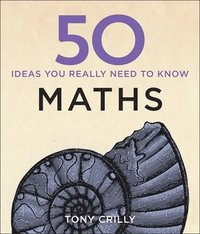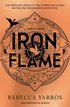Format
Inbunden (Hardback)
Språk
Engelska
Antal sidor
208
Utgivningsdatum
2014-07-31
Förlag
Quercus Publishing
Illustratör/Fotograf
50 line illustrations
Illustrationer
50 line illustrations
Dimensioner
200 x 180 x 20 mm
Vikt
526 g
ISBN
9781848667051# 50 Maths Ideas You Really Need to Know

Inbunden,  Engelska, 2014-07-31
203
• Skickas från oss inom 7-10 vardagar.
• Fri frakt över 249 kr för privatkunder i Sverige.
Kan levereras innan julafton
Finns även som
• Läs i DRM-kompatibel e-boksläsare.
47
• Läs i DRM-kompatibel e-boksläsare.
47
• Skickas inom 7-10 vardagar
141
Who invented zero? Why 60 seconds in a minute? How big is infinity? Where do parallel lines meet? And can a butterfly's wings really cause a storm on the far side of the world? In 50 Maths Ideas You Really Need to Know, Professor Tony Crilly explains in 50 clear and concise essays the mathematical concepts - ancient and modern, theoretical and practical, everyday and esoteric - that allow us to understand and shape the world around us. Packed with diagrams, examples and anecdotes, this book is the perfect overview of this often daunting but always essential subject. For once, mathematics couldn't be simpler. Contents include: Origins of mathematics, from Egyptian fractions to Roman numerals; Pi and primes, Fibonacci numbers and the golden ratio; What calculus, statistics and algebra can actually do; The very real uses of imaginary numbers; The Big Ideas of relativity, Chaos theory, Fractals, Genetics and hyperspace; The reasoning behind Sudoku and code cracking, Lotteries and gambling, Money management and compound interest; Solving of Fermat's last theorem and the million-dollar question of the Riemann hypothesis.

## Passar bra ihop

1.2. +
3.De som köpt den här boken har ofta också köpt Iron Flame av Rebecca Yarros (häftad).

## Kundrecensioner

Har du läst boken? Sätt ditt betyg »

## Övrig information

Tony Crilly is a Reader in Mathematical Sciences at Middlesex University, having previously taught at the University of Michigan, the City University in Hong Kong and the Open University. His principal research interest is the history of mathematics, and he has written and edited many works on fractals, chaos and computing. He is the author of the acclaimed biography of the English mathematician Arthur Cayley.

## Innehållsförteckning

Introduction. Zero. Number systems. Fractions. Squares and square roots. Pi e. Infinity. Imaginary numbers. Primes. Perfect numbers. Fibonacci numbers. Golden rectangles. Pascal's triangle. Algebra. Euclid's algorithm. Logic. Proof. Sets. Calculus. Constructions. Triangles. Curves. Topology. Dimension. Fractals. Chaos. The parallel postulate. Discrete geometry. Graphs. The four-colour problem. Probability. Bayes's theory. The birthday problem. Distributions. The normal curve. Connecting data. Genetics. Groups. Matrices. Codes. Advanced counting. Magic squares. Latin squares. Money mathematics. The diet problem. The travelling salesperson. Game theory. Relativity. Fermat's last theorem. The Riemann hypothesis. Glossary. Index.